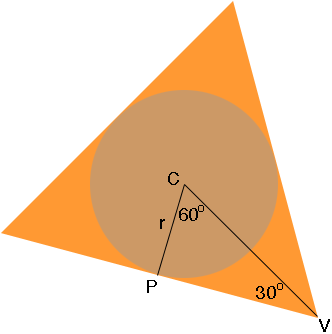SEARCH HOMEMath Central Quandaries & QueriesQuestion from Tom, a student: Hi, I need to find a container in the shape of a triangular prism that will fit four table tennis balls (this is for a math project!). These balls have a diameter of 4cm, so a radius of 2. I know that the formula for finding an incircle from a triangle is: radius of incircle = 2area of triangle / perimeter of triangle But I need to know the length of one side of the triangle from the incircle (the triangle needs to be equilateral). Can you help me find a formula for this? Thanks so much! TomHi Tom,

I drew a view of the triangular prism with a ball inside, looking down from the top. I let r be the radius of the ball.C is the centre of the circle and P is the midpoint of one of the sides. Since this is an equilateral triangle the symmetry gives us the measures of the angles. Thus triangle CPV is a 30-60-90 triangle with the length of CP being r units.

You can now use trigonometry to find the length of PV or perhaps you know the ratio of the sides of a 30-60-90 triangle.

PennyMath Central is supported by the University of Regina and The Pacific Institute for the Mathematical Sciences.